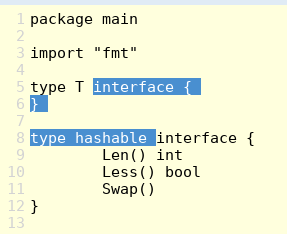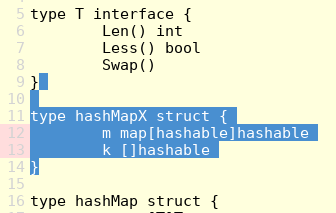# Help working with Interface

I wrote the below code that is working fine:

``````package main

import "fmt"

type T interface {
}

type hashable interface {
Len() int
Less() bool
Swap()
}

type hashMapX struct {
m map[hashable]hashable
k []hashable
}

type hashMap struct {
m map[T]T
k []T
}

// Methods required to enable sort: Len, Less, Swap > start
func (h *hashMap) Len() int {
return len(h.m)
}

func (h *hashMap) Less(i, j int) bool {
switch v := h.m[h.k[i]].(type) {
case int:
return v > h.m[h.k[j]].(int)
case float32:
return v > h.m[h.k[j]].(float32)
case float64:
return v > h.m[h.k[j]].(float64)
case string:
return v > h.m[h.k[j]].(string)
default:
return false
}
}

func (h *hashMap) Swap(i, j int) {
h.k[i], h.k[j] = h.k[j], h.k[i]
}

// Methods required to enable sort: Len, Less, Swap > end

// Build Ordered Map methods
func (h *hashMap) from(m map[T]T) hashMap {
h.m = m
h.k = make([]T, 0, len(m))
for key := range m {
h.k = append(h.k, key)
}
return *h
}

func main() {
inv := new(hashMap).from(map[T]T{"first:": 1, "second": 2})
fmt.Printf("%v", inv)
}
``````

I would like to replace `empty interface type T interface {}` using something like:

``````type T interface {
Len() int
Less() bool
Swap()
}
``````

How can I do it?

What do you want to replace? Do you want to rename `hashable` to `T`?

Want to replace the replace `empty interface type T interface {}`

``````// Want to replace
type T interface {
}

// by
type T interface {
Len() int
Less() bool
Swap()
}
``````

I don’t see anything that prevents you from doing this.

@hyousef Can you clarify what you’re trying to do, what you’ve tried, and what’s not working? Here’s what I did:

1. I took your code and put it into the Go playground.

2. Then I made `T` implement the methods from `hashable` because that’s my understanding of what you want. I did this by cutting out `T`'s implementation:3. Then I tried running the program but it didn’t work because `hashMapX` references `hashable` which I just deleted, so I deleted `hashMapX`:4. Then I tried running your program again and this time I’m getting these errors:

``````./prog.go:23:2: impossible type switch case: h.m[h.k[i]] (type T) cannot have dynamic type int (missing Len method)
./prog.go:24:25: impossible type assertion:
int does not implement T (missing Len method)
./prog.go:25:2: impossible type switch case: h.m[h.k[i]] (type T) cannot have dynamic type float32 (missing Len method)
./prog.go:26:25: impossible type assertion:
float32 does not implement T (missing Len method)
./prog.go:27:2: impossible type switch case: h.m[h.k[i]] (type T) cannot have dynamic type float64 (missing Len method)
./prog.go:28:25: impossible type assertion:
float64 does not implement T (missing Len method)
./prog.go:29:2: impossible type switch case: h.m[h.k[i]] (type T) cannot have dynamic type string (missing Len method)
./prog.go:30:25: impossible type assertion:
string does not implement T (missing Len method)
./prog.go:53:35: cannot use "first:" (type string) as type T in map key:
string does not implement T (missing Len method)
./prog.go:53:35: cannot use 1 (type int) as type T in map value:
int does not implement T (missing Len method)
./prog.go:53:35: too many errors
``````

This doesn’t work because, like the errors say, `int`, `float32`, `float64`, and `string` don’t have the methods that `T` defines.

Here I do not know what to do next because I don’t understand the goal.

`T` seems to be the type of both the keys and values of your `hashMap`. One thing you could do is create your own types that implement `T` so you can use them in your `hashMap`, but I still don’t understand the goal:

``````type Int int

func (i Int) Len() int { /* TODO */ }
func (i Int) Less() bool { /* TODO */ }
func (i Int) Swap() { /* TODO */ }
``````

But I’m not sure what it means to ge the `Len` of an `Int`, or what `Less` or `Swap` are supposed to do here.